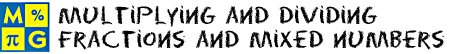Multiplying and Dividing Fractions and Mixed NumbersThis page lists the Learning Objectives for all lessons in Unit 17.

Multiplying Fractions

The student will be able to:

• Define greatest common denominator (GCF), simplify, and lowest terms.
• Recognize that the word OF means multiply.
• Describe the procedure for multiplying fractions.
• Determine the product of two or more fractions by applying the procedure above.
• Determine the product of a whole number and a fraction by applying the above procedure.
• Recognize that the result can be simplified by dividing the numerator and the denominator by their GCF, or by cancelling common factors.
• Simplify the result of each problem when necessary by using the GCF method.
• Apply the procedure above to solve word problems, and simplify the result.
• Apply multiplication procedures to complete five interactive exercises.

Multiplying Fractions By Cancelling Common Factors

The student will be able to:

• Define common factors, cancel.
• Describe the procedure for multiplying two or more fractions by dividing out common factors.
• Determine the product of two or more fractions by applying the procedure above.
• Determine the product of a whole number and a fraction by applying the procedure above.
• Recognize that the factor being divided out can appear in any numerator and any denominator.
• Recognize that dividing out common factors alleviates the need to simplify the result (unless that result is an improper fraction).
• Recognize that dividing out common factors is more efficient when working with large numbers in the numerator and the denominator.
• Apply procedures to complete five interactive exercises.

Multiplying Mixed Numbers

The student will be able to:

• Describe the procedure for multiplying mixed numbers.
• Recognize that each mixed number must be converted to an improper fraction before multiplying
• Determine the product of two or more mixed numbers by applying the procedure above.
• Simplify the result when necessary.
• Connect finding the area of real-world objects to multiplying mixed numbers.
• Recognize that in some cases, there are no common factors to divide out.
• Apply procedures to complete five interactive exercises.

Reciprocals

The student will be able to:

• Define reciprocals, inverting.
• Describe the relationship between a fraction and its reciprocal.
• Recognize that reciprocals come in pairs.
• Determine the reciprocal of a given fraction.
• Recognize that to find the reciprocal of a mixed number, you must convert it to an improper fraction first.
• Determine the reciprocal of a mixed number by converting it to an improper fraction first.
• Apply procedures to complete five interactive exercises.

Dividing Fractions

The student will be able to:

• Define divisor, and invert.
• Recognize that division indicates how many times one quantity is contained in another quantity.
• Recognize that dividing a first fraction by a second, nonzero fraction, is the same as multiplying the first fraction by the reciprocal of the second fraction.
• Describe the procedure for dividing one fraction by another.
• Recognize that the second fraction must be a nonzero number.
• Describe the process of inverting and multiplying.
• Apply reciprocal relationships to convert division of fractions into a multiplication problem.
• Apply procedures to divide fractions and simplify the result when necessary.
• Apply procedures to cancel common divisors.
• Connect real-world problems to dividing fractions.
• Apply procedures to complete five interactive exercises.

Dividing Mixed Numbers

The student will be able to:

• Describe the procedure for dividing mixed numbers.
• Recognize that each mixed number must be converted to an improper fraction before dividing.
• Apply the procedure for dividing mixed numbers, cancelling out common divisors when necessary.
• Simplify the result when necessary.
• Recognize that some problems will have no common divisors.
• Connect real-world problems to dividing mixed numbers.
• Apply procedures to complete five interactive exercises.

Solving Word Problems

The student will be able to:

• Examine real world problems involving multiplication and division of fractions and mixed numbers.
• Identify strategies for solving each problem.
• Apply strategies for solving each problem.
• Connect addition and subtraction of fractions and mixed numbers with the real word.
• Apply all concepts and procedures to complete five interactive exercises with real-world problems.

Practice Exercises

The student will be able to:

• Examine ten interactive exercises for all topics in this unit.
• Identify the concepts and procedures needed to complete each practice exercise.
• Compute all answers and solve all problems by applying appropriate concepts and procedures.
• Self-assess knowledge and skills acquired from this unit of instruction.

Challenge Exercises

The student will be able to:

• Evaluate ten challenging exercises for all topics in this unit.
• Analyze each problem to identify the given information.
• Formulate a strategy for solving each problem.
• Apply strategies to solve problems and write answers.
• Synthesize all information presented in this unit.
• Develop strong problem-solving skills and the ability to handle challenging problems.

Solutions

The student will be able to:

• Examine the solution for each exercise presented in this unit.
• Compare solutions to completed exercises.
• Identify which solutions need to be reviewed.
• Identify and evaluate incorrect exercise answers from this unit.
• Amend and label original answers.
• Identify areas of strength and weakness.
• Decide which concepts and procedures need to be reviewed from this unit.

Order your CD today for Home or SchoolOR